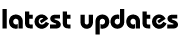Physics : Measurement Units

Measurement Units

Acre: A measure of land, 4,840 square yards (4,046 square metres).

Ampere: Unit for measuring the strength of an electric current. It is the amount of current sent by one volt through a resistance of one ohm.

Angstrom: It is the unit for measuring the wavelength of light. It is one hundred-millionth of a centimetre.

Astronomical unit: A unit of length equal to the mean radius of earth orbit. It is 149,597,870 km (92,955,800 miles).

Bar: Unit of atmospheric pressure. One bar is equal to a pressure of 106 dynes per sq cm.

Barrel: For measuring liquids. One barrel is equal to 31.1/2 gallons in US and 36 imperial gallons in Britain.

Bushel: Unit of dry measure for grain, fruit, etc. It is equal to 4 pecks or 8 gallons.

Calorie: Unit for measuring the amount of heat required to raise the temperature of one gram of water through 1°C. It is used as the unit for measuring the energy produced by food when oxidised in the body.

Carat: Unit of weight for precious stones and pearls. It is equal to about 3.17 grains troy or .2 of gram. It is also a measure of the purity of gold alloy indicating how many parts out of 24 are pure.

Coulomb: Unit for measuring the quantity of an electric current. It is the amount of electricity provided by a current of one ampere flowing for one second.

Decibel: Unit for measuring the volume of sound. It is equal to the logarithm of the ratio of the intensity of sound to the intensity of an arbitrarily chosen standard sound.

Dyne: Amount of force that causes a mass of one gram to alter its speed by one centimetre per second for each second during which the force acts. This unit of force is in CGS (metric) system.

Erg: Unit of work or energy in CGS (metric) system. It is the amount of work done by one dyne acting through a distance of one centimetre.

Farad: Electromagnetic unit of capacitance. It is equal to the amount that permits the storing of one coulomb of charge for each volt of applied potential difference.

Fathom: Unit for measuring the depth of water or the length of a rope or cable. One fathom is equal to 6 feet.

Gallon: It is a measure of liquid.

Gross: 12 dozens or 144.

Hertz: Modern unit for measurement of electromagnetic wave frequencies.

Horse power: Unit for measuring the power of motors or engines. It is equal to a rate of 33,000 foot-pounds per minute, i.e., the force required to raise 33,000 pounds at the rate of one foot per minute. HP = 746 wattsMy Great Web page